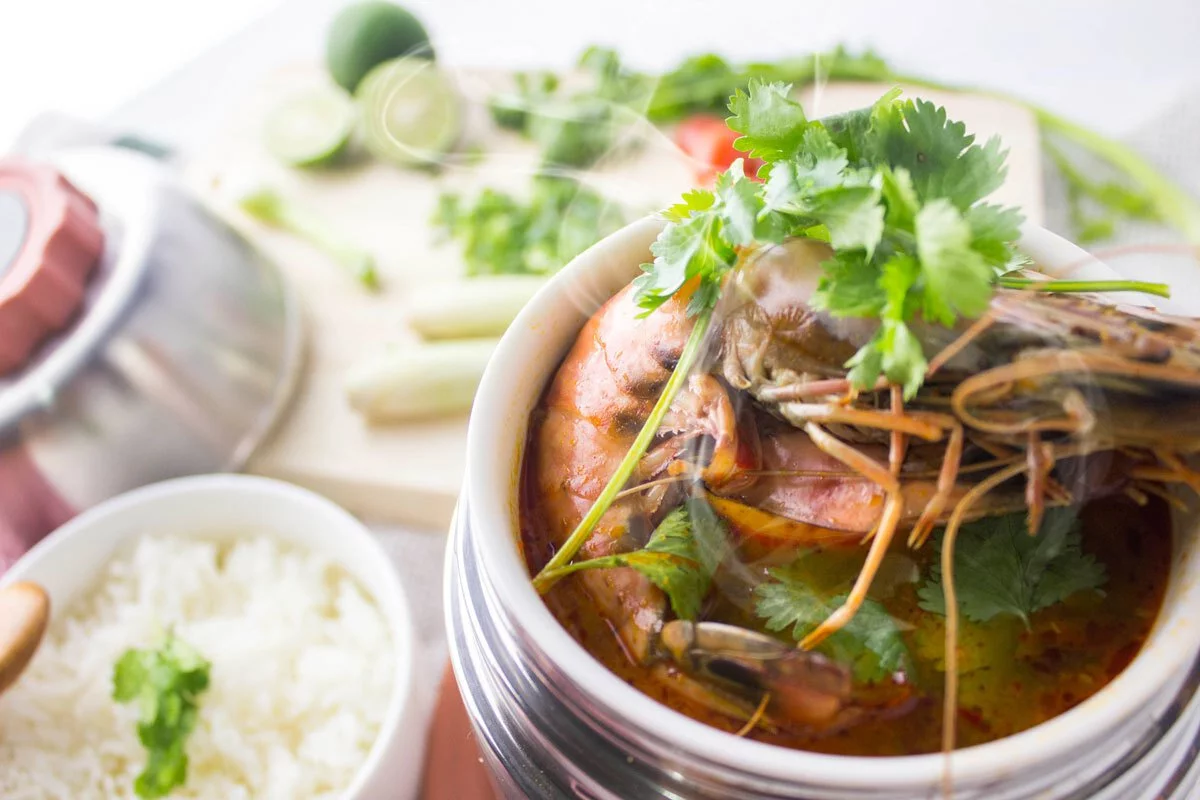• English
• 中文（繁體）
• 波多黎各
• 巴勒斯坦
• 葡萄牙
• 帕勞群島
• 巴拉圭
• 卡塔爾
• 阿森松島
• 安道爾
• 阿拉伯聯合酋長國
• 阿富汗
• 安提瓜島和巴布達
• 安圭拉
• 阿爾巴尼亞
• 亞美尼亞
• 荷屬安地列斯
• 安哥拉
• 南極洲
• 阿根廷
• 美屬薩摩亞
• 奧地利
• 留尼旺島
• 澳大利亞
• 阿魯巴
• 奧蘭群島
• 阿塞拜疆
• 羅馬尼亞
• 波斯尼亞和黑塞哥維那
• 巴巴多斯島
• 塞爾維亞
• 孟加拉
• 俄羅斯
• 比利時
• 布基納法索
• 保加利亞
• 盧旺達
• 巴林
• 布隆迪
• 貝寧
• 百慕大
• 文萊
• 玻利維亞
• 沙特阿拉伯
• 所羅門群島
• 巴西
• 塞舌爾
• 巴哈馬
• 蘇丹
• 不丹
• 瑞典
• 新加坡
• 布韋島
• 博茨瓦納
• 聖赫勒拿
• 斯洛文尼亞
• 白俄羅斯
• 斯瓦爾巴和揚馬廷
• 伯利茲
• 斯洛伐克
• 塞拉利昂
• 聖馬力諾
• 塞內加爾
• 索馬里
• 加拿大
• 蘇里南
• 科科斯群島
• 剛果民主共和國
• 聖多美和普林西比
• 中非共和國
• 薩爾瓦多
• 剛果
• 瑞士
• 科特迪瓦
• 敘利亞
• 斯威士蘭
• 庫克群島
• 智利
• 喀麥隆
• 中國
• 哥倫比亞
• 特里斯坦-達庫尼亞
• 哥斯達黎加
• 特克斯和凱科斯群島
• 乍得
• 古巴
• 法屬南部領地
• 佛得角
• 多哥
• 泰國
• 聖誕島
• 塞浦路斯
• 塔吉克斯坦
• 捷克共和國
• 托克勞
• 東帝汶
• 土庫曼斯坦
• 突尼斯
• 湯加
• 土耳其
• 特立尼達和多巴哥
• 德國
• 圖瓦盧
• 吉布提
• 坦桑尼亞
• 丹麥
• 多米尼加
• 多米尼加共和國
• 烏克蘭
• 烏干達
• 阿爾及利亞
• 美屬外島
• 美國
• 厄瓜多爾
• 愛沙尼亞
• 埃及
• 西撒哈拉
• 烏拉圭
• 烏茲別克斯坦
• 梵蒂岡
• 厄立特里亞
• 聖文森特和格林納丁斯
• 西班牙
• 埃塞俄比亞
• 委內瑞拉
• 維爾京群島，英屬
• 維爾京群島，美屬
• 越南
• 瓦努阿圖
• 芬蘭
• 斐濟
• 福克蘭群島
• 密克羅尼西亞
• 法羅群島
• 法國
• 瓦利斯和福圖納
• 加蓬
• 英國
• 薩摩亞
• 格林納達
• 喬治亞
• 法屬圭亞那
• 格恩西島
• 加納
• 直布羅陀
• 格陵蘭
• 岡比亞
• 幾內亞
• 瓜德羅普
• 赤道幾內亞
• 希臘
• 南喬治亞和南桑威奇群島
• 危地馬拉
• 關島
• 幾內亞比紹
• 圭亞那
• 中國香港特別行政區
• 赫德和麥克唐納群島
• 洪都拉斯
• 克羅地亞
• 海地
• 也門
• 匈牙利
• 印度尼西亞
• 馬約特島
• 愛爾蘭
• 以色列
• 曼島
• 印度
• 英屬印度洋領地
• 南非
• 伊拉克
• 伊朗
• 冰島
• 意大利
• 贊比亞
• 澤西島
• 津巴布韋
• 牙買加
• 約旦
• 日本
• 肯尼亞
• 吉爾吉斯斯坦
• 柬埔寨
• 基里巴斯
• 科摩羅
• 聖基茨和尼維斯
• 朝鮮
• 韓國
• 科威特
• 開曼群島
• 哈薩克斯坦
• 老撾
• 黎巴嫩
• 聖盧西亞
• 列支敦士登
• 斯里蘭卡
• 利比里亞
• 萊索托
• 立陶宛
• 盧森堡
• 拉脫維亞
• 利比亞
• 摩洛哥
• 摩納哥
• 摩爾多瓦
• 黑山
• 聖馬丁
• 馬達加斯加
• 馬紹爾群島
• 馬其頓
• 馬里
• 緬甸
• 蒙古
• 中國澳門特別行政區
• 北馬里亞納群島
• 馬提尼克
• 毛里塔尼亞
• 蒙特塞拉特
• 馬耳他
• 毛里求斯
• 馬爾代夫
• 馬拉維
• 墨西哥
• 馬來西亞
• 莫桑比克
• 納米比亞
• 新喀裡多尼亞
• 尼日爾
• 諾福克
• 尼日利亞
• 尼加拉瓜
• 荷蘭
• 挪威
• 尼泊爾
• 瑙魯
• 紐埃
• 新西蘭
• 阿曼
• 巴拿馬
• 秘魯
• 法屬波利尼西亞
• 巴布亞新幾內亞
• 菲律賓
• 巴基斯坦
• 波蘭
• 聖皮埃爾和米克隆群島
• 皮特凱恩
• HK\$ HKD
• \$ USD
• £ GBP
• EUR
• A\$ AUD
• INR
• ¥ JPY
• NT\$ TWD

# 冬陰功湯(泰式酸辣蝦湯)10隻 蝦子
6根 新鮮辣椒
6片 泰式檸檬葉
2根 香茅
6個 草菇

3片南薑

2大匙 Tom Yum醬
3又1/2匙 萊姆汁
3大匙 魚露
2大匙 奶水(淡奶)

## 作法：

1. 將蝦子剝殼後，蝦仁與蝦頭分開裝盤。
2. 取三杯量的冷水煮沸，並放入蝦頭。
3. 待蝦頭變紅色，湯成淺橘紅色便把蝦頭撈起，留下高湯。
4. 將香茅切片，與泰式檸檬葉、南薑、辣椒、草菇，以及Tom Yum醬一同加入高湯中，煮至有香氣即可。
5. 加入蝦仁、雞胸肉。 (放入後不要急著攪拌，馬上攪拌會造成蝦子腥味跑進湯裡，稍等蝦子變色50％再開始翻動，等蝦子全變色即可馬上關火。)
6. 依照個人口味加入魚露以及萊姆汁調味。
7. 最後煮好的冬陰功湯倒入Swanz的陶瓷食物保溫罐中。趁熱與米飯一同食用。